## 5.1 Background acoustic noise evaluation

06.623GPPComfort noise aspects for Enhanced Full Rate (EFR) speech traffic channelsTS

The comfort noise parameters to be encoded into a SID frame are calculated over N = 8 consecutive frames marked with VAD = 0, as follows:

The averaged LSF parameter vector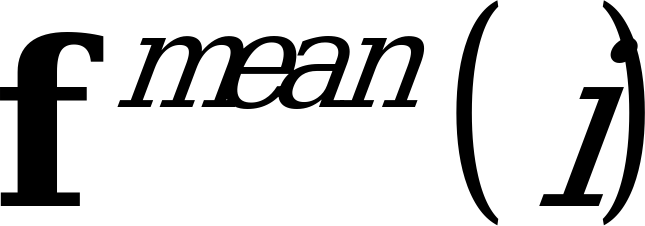of the frame i shall be computed according to the equation: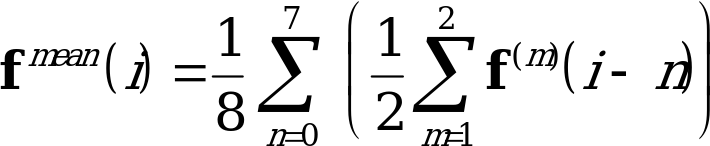(1)

where: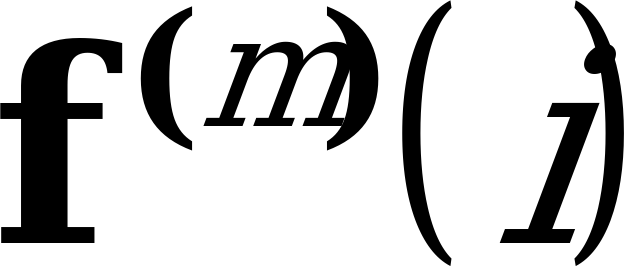is the mth (unquantized) LSF parameter vector of the current frame i (n = 0);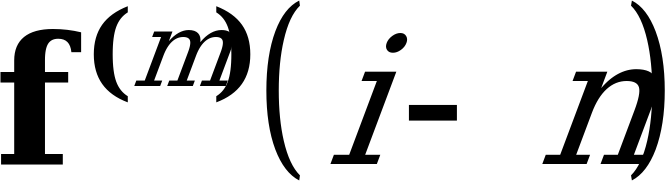is the mth (quantized or unquantized) LSF parameter vector of one of the last frames (n = 1,…, 7);

n is the averaging period index (n = 0, 1,…, 7);

m is the LSF parameter vector index within a frame (1 or 2);

i is the frame index.

NOTE: When the averaging is performed at the end of the hangover period (first SID update), all of the LSF parameter vectors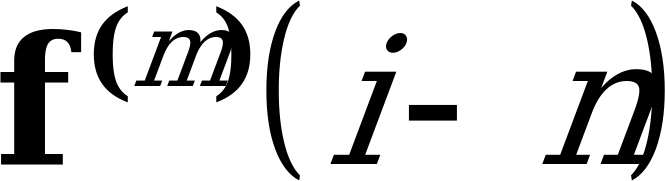of the 7 previous frames (the hangover period) have quantized values, while the LSF parameter vectors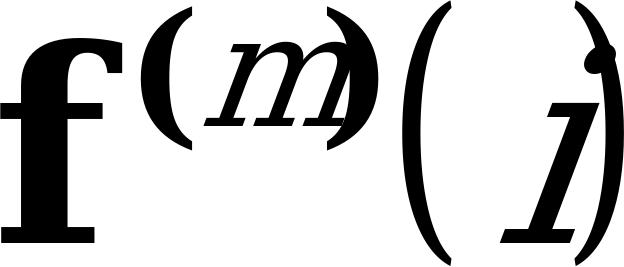of the current frame i have unquantized values. In the subsequent SID updates, the LSF parameter vectors of the SID averaging period in the frames overlapping with the hangover period have quantized values, while the parameter vectors of the more recent frames of the SID averaging period have unquantized values.

The averaged LSF parameter vector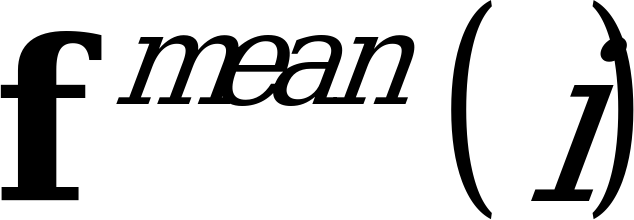of the frame i is encoded using the same encoding tables that are also used by the GSM Enhanced Full Rate speech codec for the encoding of the non-averaged LSF parameter vectors in ordinary speech encoding mode, but the quantization algorithm is modified in order to support the quantization of comfort noise. The LSF parameter prediction residual to be quantized is obtained according to the following equation: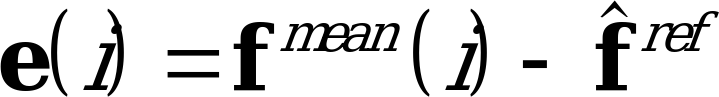(2)

where: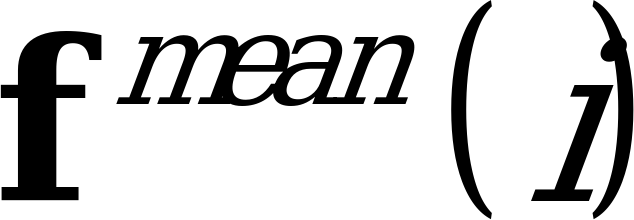is the averaged LSF parameter vector at the current frame i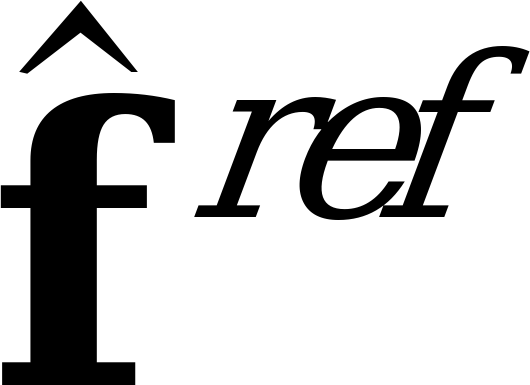is the reference LSF parameter vector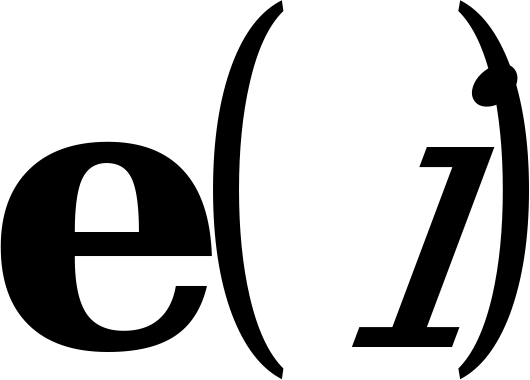is the computed LSF parameter prediction residual at the current frame i

i is the frame index;

NOTE: This prediction residual is used for both halves of the frame in the quantization algorithm. The computation of the reference LSF parameter vector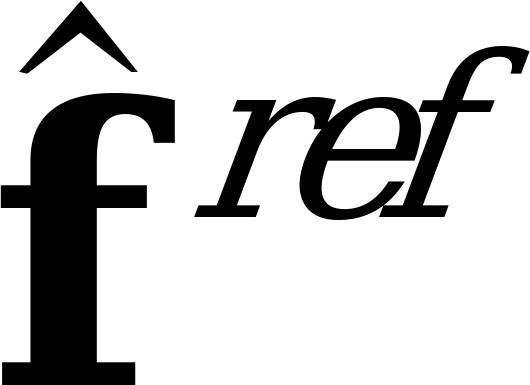is made on the basis of the quantized LSF parameters,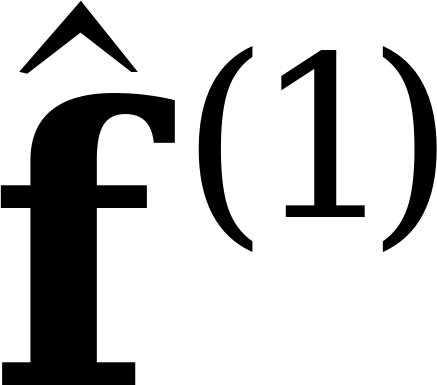and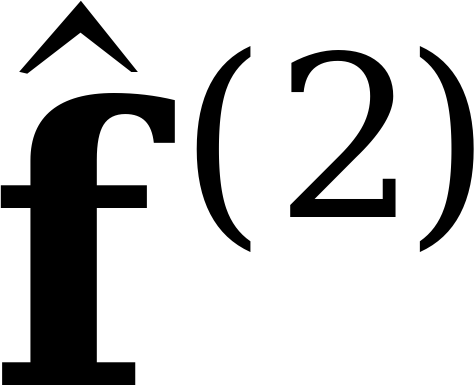, by averaging the parameters over the hangover period of 7 frames, according to the following equation:

(3)

where:

is the mth quantized LSF parameter vector of one of the frames of the hangover period
(n = 1,…, 7);

n is the hangover period frame index (n = 1,…, 7);

m is the LSF parameter index within a frame (1 or 2);

k is the frame index.

For each comfort noise insertion period, the computation of the reference LSF parameter vector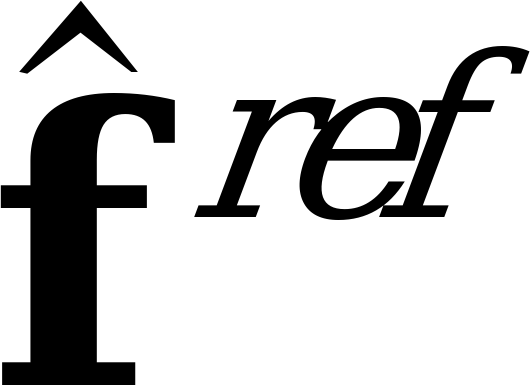is done only once at the end of the hangover period and for the rest of the comfort noise insertion periodwill be frozen. The reference LSF parameter vector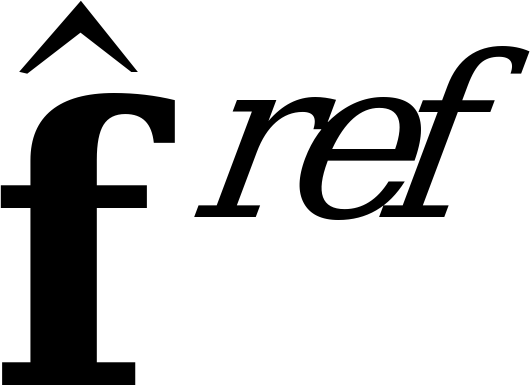is evaluated in the decoder in the same way as in the encoder, because during the hangover period the same LSF parameter vectors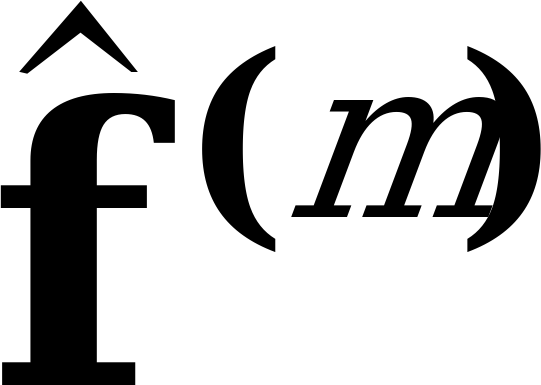are available at the encoder and decoder. An exception to this are the cases when transmission errors are severe enough to cause the parameters to become unusable, and the frame substitution procedure is activated (see GSM 06.61 ). In these cases, the modified parameters obtained from the frame substitution procedure are used instead of the received parameters.

The fixed codebook gain values shall be averaged and updated in every subframe according to the equation: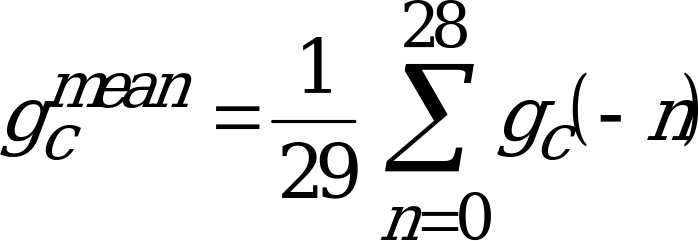(4)

where: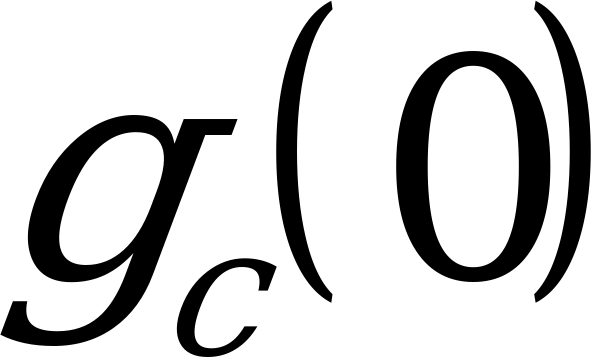is the (unquantized) fixed codebook gain in the current subframe (n=0);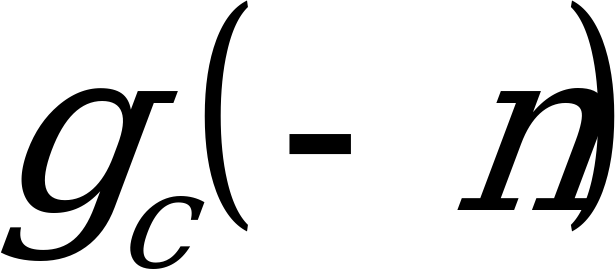is the (quantized or unquantized) fixed codebook gain in one of the past

subframes (n = 1,…, 28);

n is the averaging period index (n = 0, 1,…, 28);

NOTE: When the averaging is started at the end of the hangover period (first SID update), all of the fixed codebook gains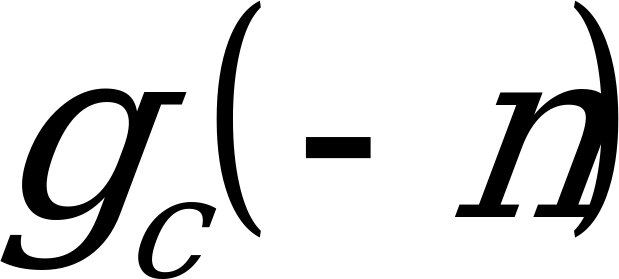of the 28 (n=1,…, 28) previous subframes (the hangover period) have quantized values, while the fixed codebook gains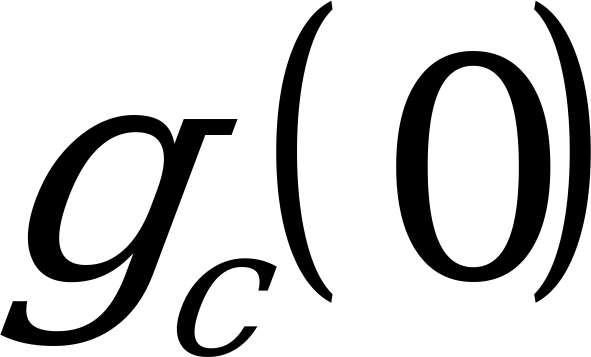of the current subframe has an unquantized value. In the subsequent SID updates, the fixed codebook gain values of the SID averaging period in the subframes overlapping with the hangover period have quantized values, while the parameter vectors of the more recent subframes of the SID averaging period have unquantized values.

Since most parts of the subframe processing clause in the encoder are switched off when the SP flag = "0" (to minimize the average complexity of the speech encoder algorithm), the unquantized fixed codebook gain is not directly available for gain averaging. Due to this, the unquantized fixed codebook gain is separately computed, based on the energy of the LP residual signal in each subframe, according to the following equation: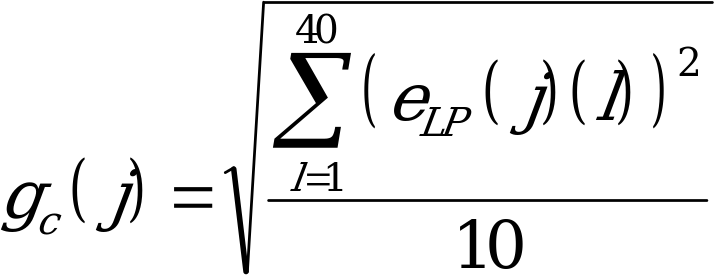(5)

where: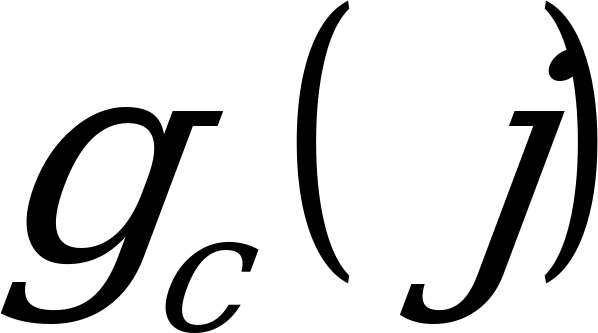is the unquantized fixed codebook gain of the current subframe j;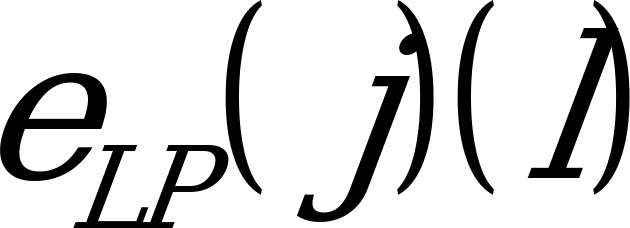is the lth sample of the LP residual in the current subframe j;

j is the subframe index (j = 1,…, 4);

l is the sample index (l = 1,…, 40).

NOTE: The computed energy of the LP residual signal is divided by the value of 10 to yield the energy for one excitation pulse, since during comfort noise generation, the subframe excitation signal (pseudo noise) has 10 non-zero samples, whose amplitudes can take values of +1 or ‑1.

The averaged fixed codebook gain value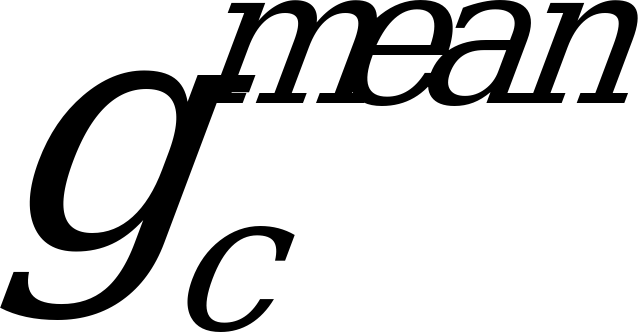of the current subframe is encoded using the non‑averaged fixed codebook gain values in ordinary speech encoding mode, but the quantization algorithm is modified in order to support comfort noise quantization. The fixed codebook gain correction factorto be quantized is obtained according to the following equation: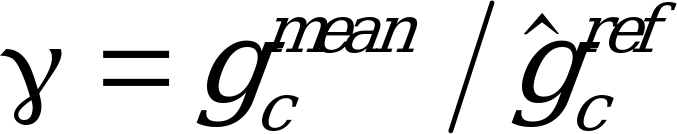(6)

where: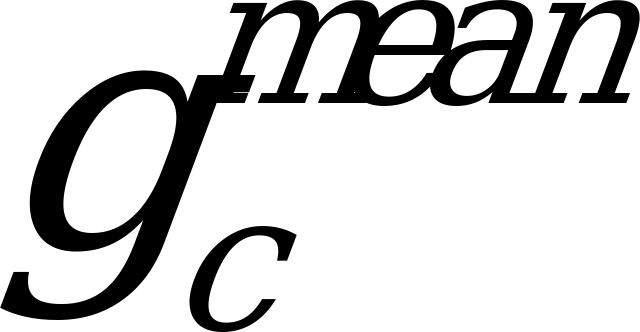is the averaged fixed codebook gain value in the current subframe;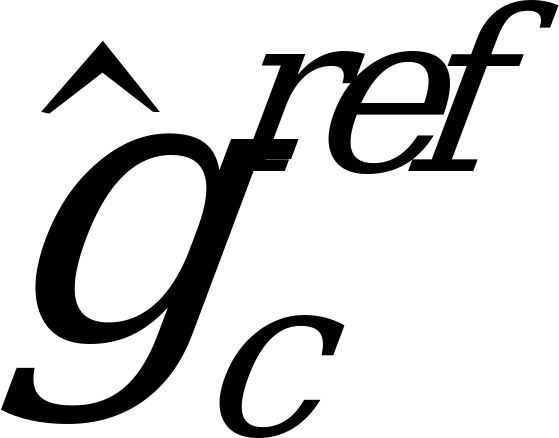is the reference fixed codebook gain;

The computation of the reference fixed codebook gainis made on the basis of the quantized fixed codebook gain parameters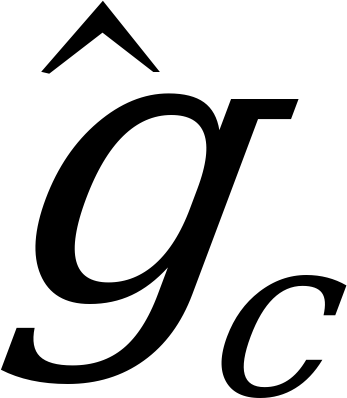, by averaging the parameter values over the hangover period of 7 frames according to the following equation:

(7)

where:

is the quantized fixed codebook gain parameter value in subframe j of one of the frames of the hangover period (n = 1,…, 7);

n is the hangover period frame index (n = 1, 2,…, 7);

k is the frame index;

j is the subframe index (j = 1,…, 4);

For each comfort noise insertion period, the computation of the reference fixed codebook gain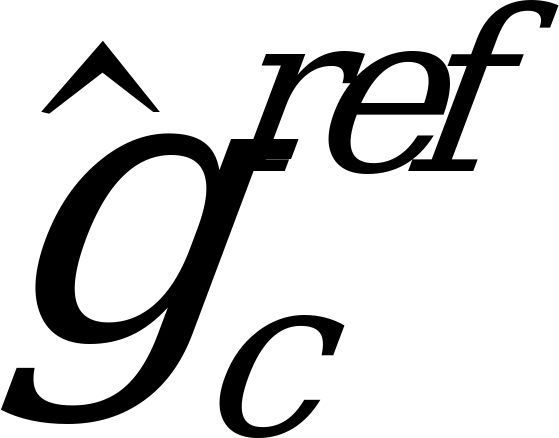is done only once at the end of the hangover period and for the rest of the comfort noise insertion periodwill be frozen. The reference fixed codebook gaincan be evaluated in the decoder in the same way as in the encoder, because during the hangover period the same quantized fixed codebook gain values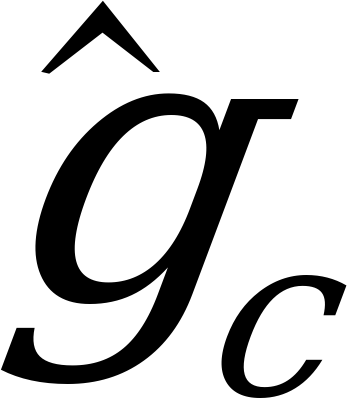are available at the encoder and decoder. An exception to this are the cases when transmission errors are severe enough to cause the parameters to become unusable, and the frame substitution procedure is activated (see GSM 06.61 ). In these cases, the modified parameters obtained from the frame substitution procedure are used instead of the received parameters.

The hangover period is defined in GSM 06.81 . It is a period added at the end of a speech burst in which no voice activity is detected (VAD flag = "0"), but the speech encoder stays for the processing of 7 speech frames in speech encoding mode (SP flag = "1"). This hangover period and the first SID frame are used for averaging the comfort noise parameters contained in the first SID frame.